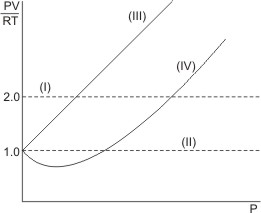## Ideal Gas Equation

Ideal Gas Equation Combining Boyle’s, Charles’ and Avogadro’s laws, we obtain:

PV = nRT - this is known as equation of state of an ideal gas (or general gas law equation). n is the number of moles of the gas and R is the gas constant. P, V and T are the pressure, volume and the absolute temperature of the gas respectively.

Plotting the graph of PV/RT over pressure, we obtain the horizontal straight lines (see below) for idea or perfect gases.

Line (I) represents 2 moles of an ideal gas, while line (II) represents 1 mole of an ideal gas. Lines (III) and (IV) represent real gases – real gases deviate from ideal behaviors at high pressures and low temperatures.

Notice that at the limit of zero pressure, all gases show perfect or ideal behavior.### Calculation Based on Ideal Gas Equation

Calculate the volume occupied by 5.0 g of carbon monoxide gas at 25 oC and 0.97 4 atm pressure. (R = 0.082 liter-atm per mole per deg, C=12, O=16).

Solution:

T = (273 + 25) = 298 K, P = 0.974 atm

n = number of moles = mass/molar mass = 5.0 /28 = 0.18 mole

PV =nRT

V = nRT/P = 0.18x0.082x298/0.974 = 4.5 liter (or dm3)

 Like This Post? Please Share!!

Copyright , All Rights Reserved Free Chemistry Online | About Us | Usage of Content | Total Disclosures | Privacy Policy